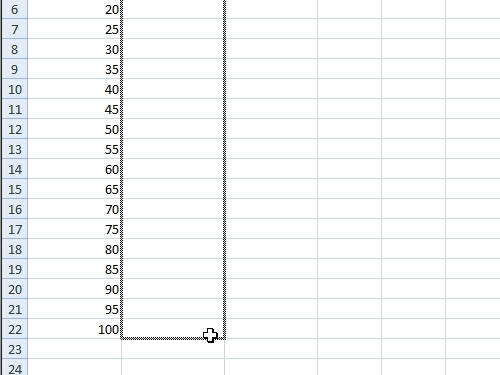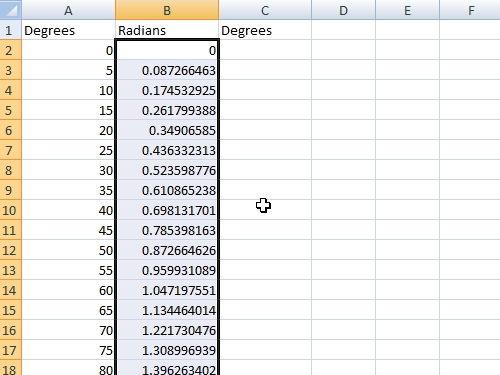## How to Convert Excel 2007 from Radians to Degrees

In this video tutorial we will show you how to convert excel from radians to degrees.

In order to convert excel from radians to degrees, open your document with degrees. To calculate radians use the following formula “A2*pi()/180”. To edit all rows with the same formula, press the left mouse button at the lower right edge of the cell, hold it and drag it down.Now to convert radians to degrees use this formula “B2*180/pi()”. Press the left mouse button, hold and drag it down to edit all rows with the same formula.